# The NLMIXED Procedure

### Modeling Assumptions and Notation

Subsections:

PROC NLMIXED operates under the following general framework for nonlinear mixed models. Assume that you have an observed data vector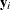for each of i subjects,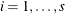. Theare assumed to be independent across i, but within-subject covariance is likely to exist because each of the elements ofis measured on the same subject. As a statistical mechanism for modeling this within-subject covariance, assume that there exist latent random-effect vectors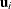of small dimension (typically one or two) that are also independent across i. Assume also that an appropriate model linkingandexists, leading to the joint probability density function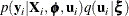where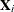is a matrix of observed explanatory variables and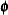and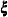are vectors of unknown parameters.

Let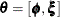and assume that it is of dimension n. Then inferences aboutare based on the marginal likelihood function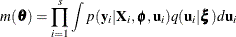In particular, the function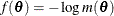is minimized overnumerically in order to estimate, and the inverse Hessian (second derivative) matrix at the estimates provides an approximate variance-covariance matrix for the estimate of. The function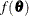is referred to both as the negative log likelihood function and as the objective function for optimization.

As an example of the preceding general framework, consider the nonlinear growth curve example in the section Getting Started: NLMIXED Procedure. Here, the conditional distribution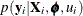is normal with mean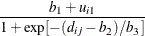and variance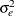; thus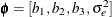. Also,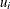is a scalar and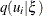is normal with mean 0 and variance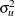; thus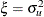.

The following additional notation is also found in this chapter. The quantity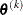refers to the parameter vector at the kth iteration, the vector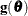refers to the gradient vector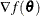, and the matrix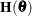refers to the Hessian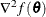. Other symbols are used to denote various constants or option values.

#### Nested Multilevel Nonlinear Mixed Models

The general framework for nested multilevel nonlinear mixed models in cases of two levels can be explained as follows. Let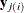be the response vector observed on subject j that is nested within subject i, where j is commonly referred as the second-level subject and i is the first-level subject. There are s first-level subjects, and each has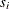second-level subjects that are nested within. An example is, which are the heights of students in class j of school i, where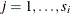for each i and. Suppose there exist latent random-effect vectors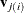and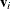of small dimensions for modeling within subject covariance. Assume also that an appropriate model that linksand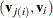exists, and if you use the notation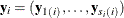,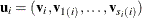, and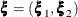, the joint density function in terms of the first-level subject can be expressed as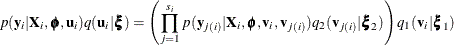As defined in the previous section, the marginal likelihood function whereisAgain, the functionis minimized overnumerically in order to estimate. Models that have more than two levels follow similar notation.